# 15 Pattern Recognition With MATCH_RECOGNIZE

This chapter provides reference and usage information about the `MATCH_RECOGNIZE` clause in Oracle Continuous Query Language (Oracle CQL). This clause and its sub-clauses perform pattern recognition in Oracle CQL queries.

Pattern matching with multiple streams in FROM clause is also supported.

This chapter includes the following sections:

## 15.1 Understanding Pattern Recognition With `MATCH_RECOGNIZE`

The `MATCH_RECOGNIZE` clause performs pattern recognition in an Oracle CQL query. This query will export (make available for inclusion in the `SELECT`) the `MEASURES` clause values for events (tuples) that satisfy the `PATTERN` clause regular expression over the `DEFINE` clause conditions.

```<query id="detectPerish"><![CDATA[
from tkrfid_ItemTempStream
MATCH_RECOGNIZE (
PARTITION BY itemId
PATTERN (A B* C)
DEFINE
A  AS  (A.temp >= 25),
B  AS  ((B.temp >= 25) and (to_timestamp(B.element_time) - to_timestamp(A.element_time) < INTERVAL "0 00:00:05.00" DAY TO SECOND)),
C  AS  (to_timestamp(C.element_time) - to_timestamp(A.element_time) >= INTERVAL "0 00:00:05.00" DAY TO SECOND)
) as its
]]></query>
```

pattern_recognition_clause::=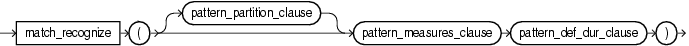pattern_def_dur_clause::=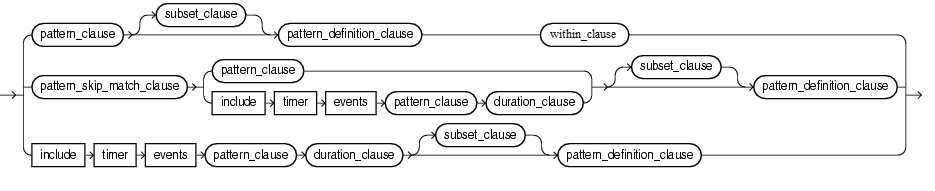Using `MATCH_RECOGNIZE`, you define conditions on the attributes of incoming events and identify these conditions by using `identifiers` called correlation variables. The previous example defines correlation variables `A`, `B`, and `C`. A sequence of consecutive events in the input stream satisfying these conditions constitutes a pattern.

The output of a `MATCH_RECOGNIZE` query is always a stream.

The principle `MATCH_RECOGNIZE` sub-clauses are:

• `MEASURES`: exports (makes available for inclusion in the `SELECT`) attribute values of events that successfully match the pattern you specify.

See MEASURES Clause.

• `PATTERN`: specifies the pattern to be matched as a regular expression over one ore more correlation variables.

See PATTERN Clause.

• `DEFINE`: specifies the condition for one or more correlation variables.

See DEFINE Clause.

To refine pattern recognition, you may use the optional `MATCH_RECOGNIZE` sub-clauses, including:

### 15.1.1 MATCH_RECOGNIZE and the WHERE Clause

In Oracle CQL (as in SQL), the `FROM` clause is evaluated before the `WHERE` clause.

Consider the following Oracle CQL query:

```SELECT ... FROM S MATCH_RECOGNIZE ( .... ) as T WHERE ...
```

In this query, the `S MATCH_RECOGNIZE ( .... ) as T` is like a subquery in the `FROM` clause and is evaluated first, before the `WHERE` clause.

Consequently, you rarely use both a `MATCH_RECOGNIZE` clause and a `WHERE` clause in the same Oracle CQL query. Instead, you typically use a view to apply the required `WHERE` clause to a stream and then select from the view in a query that applies the `MATCH_RECOGNIZE` clause.

The following example shows two views, `e1p1` and `e2p2`, each applying a `WHERE` clause to stream `S` to pre-filter the stream for the required events. The query `q` then selects from these two views and applies the `MATCH_RECOGNIZE` on this filtered stream of events.

```<view id="e1p1">
SELECT * FROM S WHERE eventName = 'E1' and path = 'P1' and statName = 'countValue'
</view>
<view id="e2p2">
SELECT * FROM S WHERE eventName = 'E2' and path = 'P2' and statName = 'countValue'
</view>

<query id="q">
SELECT
T.e1p1Stat as e1p1Stat, T.e2p2Stat as e2p2Stat
FROM
e1p1, e2p2
MATCH_RECOGNIZE(
ALL MATCHES
PATTERN(A+)
DURATION 60 MINUTES
DEFINE
A as (A.e1p1Stat < 1000 and A.e2p2Stat > 2000 and count(A) > 3)
) as T
</query>
```

### 15.1.2 Referencing Singleton and Group Matches

The `MATCH_RECOGNIZE` clause identifies the following types of matches:

• singleton: a correlation variable is a singleton if it occurs exactly once in a pattern, is not defined by a `SUBSET`, is not in the scope of an alternation, and is not quantified by a pattern quantifier.

References to such a correlation variable refer to this single event.

• group: a correlation variable is a group if it occurs in more than one pattern, is defined by a `SUBSET`, is in the scope of an alternation, or is quantified by a pattern quantifier.

References to such a correlation variable refer to this group of events.

When you reference singleton and group correlation variables in the `MEASURES` and `DEFINE` clauses, observe the following rules:

• For singleton correlation variables, you may reference individual event attributes only, not aggregates.

• For group correlation variables:

• If you reference an individual event attribute, then the value of the last event to match the correlation variable is returned.

If the correlation variable is not yet matched, `NULL` is returned. In the case of `count(A.*)`, if the correlation variable `A` is not yet matched, 0 is returned.

If the correlation variable is being referenced in a definition of the same variable (such as `DEFINE A as A.balance > 1000`), then the value of the current event is returned.

• If you reference an aggregate, then the aggregate is performed over all events that have matched the correlation variable so far.

### 15.1.3 Referencing Aggregates

You can use any built-in, Colt, or user-defined aggregate function in the `MEASURES` and `DEFINE` clause of a `MATCH_RECOGNIZE` query.

When using aggregate functions, consider the following:

#### 15.1.3.1 Running Aggregates and Final Aggregates

In the `DEFINE` clause, any aggregate function on a correlation variable `X` is a running aggregate: that is, the aggregate includes all preceding matches of `X` up to and including the current match. If the correlation variable `X` has been completely matched so far, then the aggregate is final, otherwise it is running.

In the `MEASURES` clause, because it is evaluated after the match has been found, all aggregates are final because they are computed over the final match.

When using a `SUBSET` clause, be aware of the fact that you may inadvertently imply a running aggregate.

```...
PATTERN (X+ Y+)
SUBSET Z = (X, Y)
DEFINE
X AS X.price > 100,
Y AS sum(Z.price) < 1000
...
```

Because correlation variable `Z` involves `Y`, the definition of `Y` involves a running aggregate on `Y`.

#### 15.1.3.2 Operating on the Same Correlation Variable

In both the `MEASURES` and `DEFINE` clause, you may only apply an aggregate function to attributes of the same correlation variable.

For example: the use of aggregate function `correlation`.

```...
MEASURES xycorr AS correlation(X.price, Y.price)
PATTERN (X+ Y+)
DEFINE
X AS X.price <= 10,
Y AS Y.price > 10
...
```

The `correlation` aggregate function may not operate on more than one correlation variable.

#### 15.1.3.3 Referencing Variables That Have not Been Matched Yet

In the `DEFINE` clause, you may reference a correlation variable that has not been matched yet. However, you should use caution when doing so.

```PATTERN (X+ Y+)
DEFINE
X AS count(Y.*) >= 3
Y AS Y.price > 10,
```

Although this syntax is legal, note that in this particular example, the pattern will never match because at the time `X` is matched, `Y` has not yet been matched, and `count(Y.*)` is 0.

To implement the desired behavior ("Match when the price of `Y` has been greater than 10, 3 or more times in a row"), implement this pattern.

```PATTERN (Y+ X+)
DEFINE
Y AS Y.price > 10,
X AS count(Y.*) >= 3
```

#### 15.1.3.4 Referencing Attributes not Qualified by Correlation Variable

In the `DEFINE` clause, if you apply an aggregate function to an event attribute not qualified by correlation variable, the aggregate is a running aggregate.

```PATTERN ((RISE FALL)+)
DEFINE
RISE AS count(RISE.*) = 1 or RISE.price > FALL.price,
FALL AS FALL.price < RISE.price and count(*) > 1000
```

This query detects a pattern in which a price alternately goes up and down, for as long as possible, but for at least more than 1000 matches.

#### 15.1.3.5 Using count With *, identifier.*, and identifier.attr

The built-in aggregate function `count` has syntax: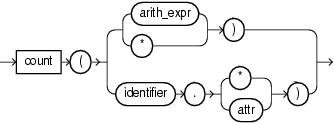The return value of `count` depends on the argument as Table 15-1 shows.

Table 15-1 Return Values for count Aggregate Function

Input Argument Return Value

`arith_expr`

The number of tuples where `arith_expr` is not `NULL`.

`*`

The number of tuples matching all the correlation variables in the pattern, including duplicates and nulls.

`identifier``.*`

The number of all tuples that match the correlation variable `identifier`, including duplicates and nulls.

Note the following:

• `count(A.*) = 1` is true for the first event that matches `A`.

• `count(A.*) = 0` is true while `A` has not been matched yet.

`identifier``.``attr`

The number of tuples that match correlation variable `identifier`, where `attr` is not `NULL`.

Assume that the schema of `S` includes attributes `account` and `balance`. This query returns an event for each `account` that has not received 3 or more events in 60 minutes.

```select
T.account,
T.Atime
FROM S
MATCH_RECOGNIZE(
PARTITION BY account
MEASURES
A.account has account
A.ELEMENT_TIME as Atime
ALL MATCHES
INCLUDE TIMER EVENTS
PATTERN (A+)
DURATION 60 MINUTES
DEFINE
A AS count(A.*) < 3
) as T
```

The `PATTERN (A+)` specifies the pattern "Match `A` one or more times".

The `DEFINE` clause specifies the condition:

```A AS count(A.*) < 3
```

This condition for `A` places no restrictions on input tuples (such as `A.balance > 1000`). The only restrictions are imposed by the `PARTITION BY account` and `DURATION 60 MINUTES` clauses. In the `DEFINE` clause, the `A.*` means, "Match all input tuples for the group `A+`". This group includes the one or more input tuples with a particular `account` received in the 60 minutes starting with the first input tuple. The `count(A.*)` is a running aggregate that returns the total number of events in this group.

If the `DEFINE` clause specifies the condition:

```A AS A.balance > 1000 and count(A.*) < 3
```

Then `A.*` still means "Match all input tuples for the group `A+`". In this case, this group includes the one or more input tuples with a particular `account` received in the 60 minutes starting with the first input tuple and with `balance > 1000`.

In contrast:

• `count(*)` means "The number of tuples matching all the correlation variables in the pattern, including duplicates and nulls."

• `count(A.balance)` means "The number of all tuples that match the correlation variable `A` where the `balance` is not `NULL`".

#### 15.1.3.6 Using `first` and `last`

Use the `first` and `last` built-in aggregate functions to access event attributes of the first or last event match, respectively:

`first` returns the value of the first match of a group in the order defined by the `ORDER BY` clause or the default order.

`last` returns the value of the last match of a group in the order defined by the `ORDER BY` clause or the default order.

The `first` and `last` functions accept an optional non-negative, constant integer argument (`N`) that indicates the offset following the first and the offset preceding the last match of the variable, respectively. If you specify this offset, the `first` function returns the `N`-th matching event following the first match and the `last` function returns the `N`-th matching event preceding the last match. If the offset does not fall within the match of the variable, the `first` and `last` functions return `NULL`.

### 15.1.4 Using `prev`

Use the `prev` built-in single-row function to access event attributes of a previous event match. If there is no previous event match, the `prev` function returns `NULL`.

The `prev` function accepts an optional non-negative, constant integer argument (`N`) that indicates the offset to a previous match. If you specify this offset, the `prev` function returns the `N`-th matching event preceding the current match. If there is no such previous match, the `prev` functions returns `NULL`.

When you use the `prev` function in the `DEFINE` clause, this function may only access the currently defined correlation variable.

For example: the correlation variable definition:

```Y AS Y.price < prev(Y.price, 2)
```

However, the correlation variable definition is invalid because while defining correlation variable `Y`, it references correlation variable `X` inside the `prev` function.

```Y AS Y.price < prev(X.price, 2)
```

## 15.2`MEASURES` Clause

The `MEASURES` clause exports (makes available for inclusion in the `SELECT`) attribute values of events that successfully match the pattern you specify.

You may specify expressions over correlation variables that reference partition attributes, order by attributes, singleton variables and aggregates on group variables, and aggregates on the attributes of the stream that is the source of the `MATCH_RECOGNIZE` clause.

You qualify attribute values by correlation variable to export the value of the attribute for the event that matches the correlation variable's condition. For example, within the `MEASURES` clause, `A.c1` refers to the value of event attribute `c1`:

• In the tuple that last matched the condition corresponding to correlation variable `A`, if `A` is specified in the `DEFINE` clause.

• In the last processed tuple, if `A` is not specified in the `DEFINE` clause.

This is because if `A` is not specified in the `DEFINE` clause, then `A` is considered as `TRUE` always. So effectively all the tuples in the input match to `A`.

You may include in the `SELECT` statement only attributes you specify in the `MEASURES` clause.

Figure 15-1 pattern_measures_clause::=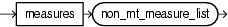non_mt_measure_list::=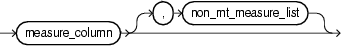measure_column::=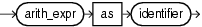The `pattern_measures_clause` is:

```MEASURES
A.itemId as itemId
```

This section describes:

### 15.2.1 Functions Over Correlation Variables in the MEASURES Clause

In the `MEASURES` clause, you may apply any single-row or aggregate function to the attributes of events that match a condition.

The following example applies the `last` function over correlation variable `Z.c1` in the `MEASURES` clause.

```<query id="tkpattern_q41"><![CDATA[
select
T.firstW, T.lastZ
from
tkpattern_S11
MATCH_RECOGNIZE (
MEASURES A.c1 as firstW, last(Z.c1) as lastZ
ALL MATCHES
PATTERN(A W+ X+ Y+ Z+)
DEFINE
W as W.c2 < prev(W.c2),
X as X.c2 > prev(X.c2),
Y as Y.c2 < prev(Y.c2),
Z as Z.c2 > prev(Z.c2)
) as T
]]></query>
```

Note the following in the `MEASURES` clause:

• `A.c1` will export the value of `c1` in the first and only the first event that the query processes because:

• `A` is not specified in the `DEFINE` clause, therefor it is always true.

• `A` has no pattern quantifiers, therefor it will match exactly once.

• The built-in aggregate function `last` will export the value of `c1` in the last event that matched `Z` at the time the `PATTERN` clause was satisfied.

## 15.3 PATTERN Clause

The `PATTERN` clause specifies the pattern to be matched as a regular expression over one ore more correlation variables.

Incoming events must match these conditions in the order given (from left to right).

The regular expression may contain correlation variables that are:

• Defined in the `DEFINE` clause: such correlation variables are considered true only if their condition definition evaluates to `TRUE`.

See DEFINE Clause.

• Not defined in the `DEFINE` clause: such correlation variables are considered as always `TRUE`; that is, they match every input.

Figure 15-2 pattern_clause::=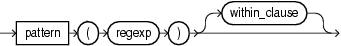This section describes:

### 15.3.1 Pattern Quantifiers and Regular Expressions

You express the pattern as a regular expression composed of correlation variables and pattern quantifiers.

regexp::=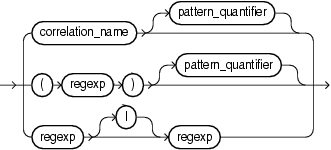correlation_name::=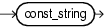pattern_quantifier::=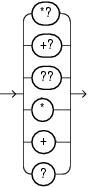Table 15-2 lists the pattern quantifiers (pattern_quantifier::=) Oracle CQL supports.

Table 15-2 MATCH_RECOGNIZE Pattern Quantifiers

Maximal Minimal Description

`*`

`*?`

0 or more times

`+`

`+?`

1 or more times.

`?`

`??`

0 or 1 time.

None

None

An unquantified pattern, such as `A`, is assumed to have a quantifier that requires exactly 1 match.

Use the pattern quantifiers to specify the pattern as a regular expression, such as `A*` or `A+?`.

The one-character pattern quantifiers are maximal or "greedy"; they will attempt to match as many instances of the regular expression on which they are applied as possible.

The two-character pattern quantifiers are minimal or "reluctant"; they will attempt to match as few instances of the regular expression on which they are applied as possible.

Consider the following `pattern_clause`:

```PATTERN (A B* C)
```

This pattern clause means a pattern match will be recognized when the following conditions are met by consecutive incoming input tuples:

1. Exactly one tuple matches the condition that defines correlation variable `A`, followed by

2. Zero or more tuples that match the correlation variable `B`, followed by

3. Exactly one tuple that matches correlation variable `C`.

While in state 2, if a tuple arrives that matches both the correlation variables `B` and `C` (since it satisfies the defining conditions of both of them) then as the quantifier `*` for `B` is greedy that tuple will be considered to have matched `B` instead of `C`. Thus due to the greedy property `B` gets preference over `C` and we match a greater number of `B`. Had the pattern expression be `A B*? C`, one that uses a lazy or reluctant quantifier over `B`, then a tuple matching both `B` and `C` will be treated as matching `C` only. Thus `C` would get preference over `B` and we will match fewer `B`.

### 15.3.2 Grouping and Alternation in the PATTERN Clause

As shown in the `regexp_grp_alt` syntax, you can use:

• open and close round brackets (`(` and `)`) to group correlation variables

• alternation operators (`|`) to match either one correlation variable (or group of correlation variables) or another

regexp_grp_alt::=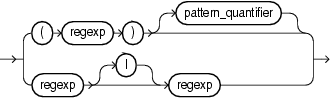Consider the following `pattern_clause`:

```PATTERN (A+ B+)
```

This means "A one or more times followed by B one or more times".

You can group correlation variables. For example:

```PATTERN (A+ (C+ B+)*)
```

This means "A one or more times followed by zero or more occurrences of C one or more times and B one or more times".

Using the `PATTERN` clause alternation operator (`|`), you can refine the sense of the `pattern_clause`. For example:

```PATTERN (A+ | B+)
```

This means "A one or more times or B one or more times, whichever comes first".

Similarly, you can both group correlation variables and use the alternation operator. For example:

```PATTERN (A+ (C+ | B+))
```

This means "A one or more times followed by either C one or more times or B one or more times, whichever comes first".

To match every permutation you can use:

```PATTERN ((A B) | (B A))
```

This means "A followed by B or B followed by A, which ever comes first".

## 15.4`DEFINE` Clause

The `DEFINE` clause specifies the boolean condition for each correlation variable.

You may specify any logical or arithmetic expression and apply any single-row or aggregate function to the attributes of events that match a condition.

On receiving a new tuple from the base stream, the conditions of the correlation variables that are relevant at that point in time are evaluated. A tuple is said to have matched a correlation variable if it satisfies its defining condition. A particular input can match zero, one, or more correlation variables. The relevant conditions to be evaluated on receiving an input are determined by logic governed by the `PATTERN` clause regular expression and the state in pattern recognition process that we have reached after processing the earlier inputs.

The condition can refer to any of the attributes of the schema of the stream or view that evaluates to a stream on which the `MATCH_RECOGNIZE` clause is being applied.

A correlation variable in the `PATTERN` clause need not be specified in the `DEFINE` clause: the default for such a correlation variable is a predicate that is always true. Such a correlation variable matches every event. It is an error to specify a correlation variable in the `DEFINE` clause which is not used in a `PATTERN` clause

No correlation variable defined by a `SUBSET` clause may be defined in the `DEFINE` clause.

Figure 15-3 pattern_definition_clause::=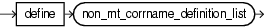Figure 15-4 non_mt_corrname_definition_list::=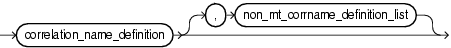Figure 15-5 correlation_name_definition::=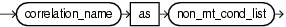This section describes:

### 15.4.1 Functions Over Correlation Variables in the DEFINE Clause

You can use functions over the correlation variables while defining them.

The following example applies the `to_timestamp` function to correlation variables.

```...
PATTERN (A B* C)
DEFINE
A  AS  (A.temp >= 25),
B  AS  ((B.temp >= 25) and (to_timestamp(B.element_time) - to_timestamp(A.element_time) < INTERVAL "0 00:00:05.00" DAY TO SECOND)),
C  AS  (to_timestamp(C.element_time) - to_timestamp(A.element_time) >= INTERVAL "0 00:00:05.00" DAY TO SECOND)
...
```

The following example applies the `count` function to correlation variable `B` to count the number of times its definition was satisfied. A match is recognized when `totalCountValue` is less than 1000 two or more times in 30 minutes.

```...
MATCH_RECOGNIZE(
...
PATTERN(B*)
DURATION 30 MINUTES
DEFINE
B as (B.totalCountValue < 1000 and count(B.*) >= 2)
...
```

### 15.4.2 Referencing Attributes in the DEFINE Clause

You can refer to the attributes of a base stream:

• Without a correlation variable: `c1 < 20`.

• With a correlation variable: `A.c1 < 20`.

When you refer to the attributes without a correlation variable, a tuple that last matched any of the correlation variables is consulted for evaluation.

Consider the following definitions:

• `DEFINE A as c1 < 20`

• `DEFINE A as A.c1 < 20`

Both refer to `c1` in the same tuple which is the latest input tuple. This is because on receiving an input we evaluate the condition of a correlation variable assuming that the latest input matches that correlation variable.

If you specify a correlation name that is not defined in the `DEFINE` clause, it is considered to be true for every input.

The correlation variable `A` appears in the `PATTERN` clause but is not specified in the `DEFINE` clause. This means the correlation name `A` is true for every input. It is an error to define a correlation name which is not used in a `PATTERN` clause.

```<query id="q"><![CDATA[
SELECT
T.firstW,
T.lastZ
FROM
S2
MATCH_RECOGNIZE (
MEASURES
A.c1 as firstW,
last(Z) as lastZ
PATTERN(A W+ X+ Y+ Z+)
DEFINE
W as W.c2 < prev(W.c2),
X as X.c2 > prev(X.c2),
Y as Y.c2 < prev(Y.c2),
Z as Z.c2 > prev(Z.c2)
) as T
]]></query>
```

### 15.4.3 Referencing One Correlation Variable From Another in the DEFINE Clause

A definition of one correlation variable can refer to another correlation variable. Consider the query:

```...
Select
a_firsttime, d_lasttime, b_avgprice, d_avgprice
FROM
S
MATCH_RECOGNIZE (
PARTITION BY symbol
MEASURES
first(a.time) as a_firsttime,
last(d.time) as d_lasttime,
avg(b.price) as b_avgprice,
avg(d.price) as d_avgprice
PATTERN (A B+ C+ D)
DEFINE
A as A.price > 100,
B as B.price > A.price,
C as C.price < avg(B.price),
D as D.price > prev(D.price)
)
...
```

Note the following:

• Because correlation variable `A` defines a single attribute, `B` can refer to this single attribute.

• Because `B` defines more than one attribute, `C` cannot reference a single attribute of `B`. In this case, `C` may only reference an aggregate of `B`.

• `D` is defined in terms of itself: in this case, you may refer to a single attribute or an aggregate. In this example, the `prev` function is used to access the match of `D` prior to the current match.

## 15.5 PARTITION BY Clause

Use this optional clause to specify the stream attributes by which a `MATCH_RECOGNIZE` clause should partition its results.

Without a `PARTITION BY` clause, all stream attributes belong to the same partition.

Figure 15-6 pattern_partition_clause::=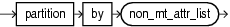The `pattern_partition_clause` is:

```PARTITION BY
itemId
```

The partition by clause in pattern means the input stream is logically divided based on the attributes mentioned in the partition list and pattern matching is done within a partition.

Consider a stream `S` with schema `(c1 integer, c2 integer)` with the input data.

```     c1  c2
1000 10, 1
2000 10, 2
3000 20, 2
4000 20, 1
```

Consider the `MATCH_RECOGNIZE` query.

```select T.p1, T.p2, T.p3 from S MATCH_RECOGNIZE(
MEASURES
A.ELEMENT_TIME as p1,
B.ELEMENT_TIME as p2
B.c2 as p3
PATTERN (A B)
DEFINE
A as A.c1 = 10,
B as B.c1 = 20
) as T
```

This query would output the following:

```3000:+ 2000, 3000, 2
```

If we add `PARTITION BY c2` to the query, then the output would change to:

```3000:+ 2000, 3000, 2
4000:+ 1000, 4000, 1
```

This is because by adding the `PARTITION BY` clause, matches are done within partition only. Tuples at 1000 and 4000 belong to one partition and tuples at 2000 and 3000 belong to another partition owing to the value of `c2` attribute in them. In the first partition `A` matches tuple at 1000 and `B` matches tuple at 4000. Even though a tuple at 3000 matches the `B` definition, it is not presented as a match for the first partition since that tuple belongs to different partition.

## 15.6 ALL MATCHES Clause

Use this optional clause to configure Oracle Event Processing to match overlapping patterns.

With the `ALL MATCHES` clause, Oracle Event Processing finds all possible matches. Matches may overlap and may start at the same event. In this case, there is no distinction between greedy and reluctant pattern quantifiers. For example, the following pattern:

```ALL MATCHES
PATTERN (A* B)
```

produces the same result as:

```ALL MATCHES
PATTERN (A*? B)
```

Without the `ALL MATCHES` clause, overlapping matches are not returned, and quantifiers such as the asterisk determine which among a set of candidate (and overlapping) matches is the preferred one for output. The rest of the overlapping matches are discarded.

Figure 15-7 pattern_skip_match_clause::=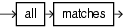Consider the query `tkpattern_q41` that uses `ALL MATCHES` and the data stream `tkpattern_S11`. Stream `tkpattern_S11` has schema `(c1 integer, c2 integer)`. The query returns the stream.

The query `tkpattern_q41` will report a match when the input stream values, when plotted, form the shape of the English letter W. The relation shows an example of overlapping instances of this W-pattern match.

There are two types of overlapping pattern instances:

• Total: Example of total overlapping: Rows from time 3000-9000 and 4000-9000 in the input, both match the given pattern expression. Here the longest one (3000-9000) will be preferred if `ALL MATCHES` clause is not present.

• Partial: Example of Partial overlapping: Rows from time 12000-21000 and 16000-23000 in the input, both match the given pattern expression. Here the one which appears earlier is preferred when `ALL MATCHES` clause is not present. This is because when `ALL MATCHES` clause is omitted, we start looking for the next instance of pattern match at a tuple which is next to the last tuple in the previous matched instance of the pattern.

```<query id="tkpattern_q41"><![CDATA[
select
T.firstW, T.lastZ
from
tkpattern_S11
MATCH_RECOGNIZE (
MEASURES A.c1 as firstW, last(Z.c1) as lastZ
ALL MATCHES
PATTERN(A W+ X+ Y+ Z+)
DEFINE
W as W.c2 < prev(W.c2),
X as X.c2 > prev(X.c2),
Y as Y.c2 < prev(Y.c2),
Z as Z.c2 > prev(Z.c2)
) as T
]]></query>
```
```Timestamp   Tuple
1000         1,8
2000         2,8
3000         3,8
4000         4,6
5000         5,3
6000         6,7
7000         7,6
8000         8,2
9000         9,6
10000        10,2
11000        11,9
12000        12,9
13000        13,8
14000        14,5
15000        15,0
16000        16,9
17000        17,2
18000        18,0
19000        19,2
20000        20,3
21000        21,8
22000        22,5
23000        23,9
24000        24,9
25000        25,4
26000        26,7
27000        27,2
28000        28,8
29000        29,0
30000        30,4
31000        31,4
32000        32,7
33000        33,8
34000        34,6
35000        35,4
36000        36,5
37000        37,1
38000        38,7
39000        39,5
40000        40,8
41000        41,6
42000        42,6
43000        43,0
44000        44,6
45000        45,8
46000        46,4
47000        47,3
48000        48,8
49000        49,2
50000        50,5
51000        51,3
52000        52,3
53000        53,9
54000        54,8
55000        55,5
56000        56,5
57000        57,9
58000        58,7
59000        59,3
60000        60,3
```
```Timestamp   Tuple Kind  Tuple
9000:      +      3,9
9000:      +      4,9
11000:      +      6,11
11000:      +      7,11
19000:      +     12,19
19000:      +     13,19
19000:      +     14,19
20000:      +     12,20
20000:      +     13,20
20000:      +     14,20
21000:      +     12,21
21000:      +     13,21
21000:      +     14,21
23000:      +     16,23
23000:      +     17,23
28000:      +     24,28
30000:      +     26,30
38000:      +     33,38
38000:      +     34,38
40000:      +     36,40
48000:      +     42,48
50000:      +     45,50
50000:      +     46,50
```

The `ALL MATCHES` clause reports all the matched pattern instances on receiving a particular input. For example, at time 20000, all of the tuples `{12,20}`, `{13,20}`, and `{14,20}` are output.

## 15.7 WITHIN Clause

The `WITHIN` clause is an optional clause that outputs a `pattern_clause` match if and only if the match occurs within the specified time duration.

Figure 15-8 within_clause::=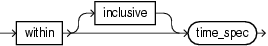That is, if and only if:

```TL - TF < WD
```

Where:

• `TL` - Timestamp of last event matching the pattern.

• `TF` - Timestamp of first event matching the pattern.

• `WD` - Duration specified in the `WITHIN` clause.

The `WITHIN INCLUSIVE` clause tries to match events at the boundary case as well. That is, it outputs a match if and only if:

```TL - TF <= WD
```

If the match completes within the specified time duration, then the event is output as soon as it happens. That is, if the match can be output, it is output with the timestamp at which it completes. The `WITHIN` clause does not wait for the time duration to expire as the `DURATION` clause does.

When the `WITHIN` clause duration expires, it discards any potential candidate matches which are incomplete.

Note:

You cannot use a `WITHIN` clause with a `DURATION` clause. For more information, see DURATION Clause.

## 15.8 DURATION Clause

The `DURATION` clause is an optional clause that you should use only when writing a query involving non-event detection. Non-event detection is the detection of a situation when a certain event which should have occurred in a particular time limit does not occur in that time frame.

Figure 15-9 duration_clause::=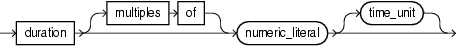Using this clause, a match is reported only when the regular expression in the `PATTERN` clause is matched completely and no other event or input arrives until the duration specified in the `DURATION` clause expires. The duration is measured from the time of arrival of the first event in the pattern match.

You must use the `INCLUDE TIMER EVENTS` clause when using the `DURATION` clause. For more information, see INCLUDE TIMER EVENTS Clause.

This section describes:

Note:

You cannot use a `DURATION` clause with a `WITHIN` clause. For more information, see WITHIN Clause.

### 15.8.1 Fixed Duration Non-Event Detection

The duration can be specified as a constant value, such as 10. Optionally, you may specify a time unit such as seconds or minutes (see Figure 7-11); the default time unit is seconds.

Consider the query `tkpattern_q59` that uses `DURATION 10` to specify a delay of 10 s (10000 ms) and the data stream `tkpattern_S19`. Stream `tkpattern_S19` has schema `(c1 integer)`. The query returns the stream.

```<query id="BBAQuery"><![CDATA[
select
T.p1, T.p2
from
tkpattern_S19
MATCH_RECOGNIZE (
MEASURES A.c1 as p1, B.c1 as p2
include timer events
PATTERN(A B*)
duration 10
DEFINE A as A.c1 = 10, B as B.c1 != A.c1
) as T
]]></query>
```
```Timestamp   Tuple
1000        10
4000        22
6000       444
7000        83
9000        88
11000        12
11000        22
11000        15
12000        13
15000        10
27000        11
28000        10
30000        18
40000        10
44000        19
52000        10
h 100000
```
```Timestamp   Tuple Kind  Tuple
11000:      +           10,88
25000:      +           10,
38000:      +           10,18
50000:      +           10,19
62000:      +           10,
```

The tuple at time 1000 matches `A`.

Since the duration is 10 we output a match as soon as input at time `1000+10000=11000` is received (the one with the value 12). Since the sequence of tuples from 1000 through 9000 match the pattern `AB*` and nothing else a match is reported as soon as input at time 11000 is received.

The next match starts at 15000 with the tuple at that time matching `A`. The next tuple that arrives is at 27000. So here also we have tuples satisfying pattern `AB*` and nothing else and hence a match is reported at time `15000+10000=25000`. Further output is generated by following similar logic.

### 15.8.2 Recurring Non-Event Detection

When you specify a `MULTIPLES OF` clause, it indicates recurring non-event detection. In this case an output is sent at the multiples of duration value as long as there is no event after the pattern matches completely.

Consider the query `tkpattern_q75` that uses `DURATION MULTIPLES OF 10` to specify a delay of 10 s (10000 ms) and the data stream `tkpattern_S23`. Stream `tkpattern_S23` has schema `(c1 integer)`. The query returns the stream.

```<query id="tkpattern_q75"><![CDATA[
select
T.p1, T.p2, T.p3
from
tkpattern_S23
MATCH_RECOGNIZE (
MEASURES A.c1 as p1, B.c1 as p2, sum(B.c1) as p3
ALL MATCHES
include timer events
PATTERN(A B*)
duration multiples of 10
DEFINE A as A.c1 = 10, B as B.c1 != A.c1
) as T
]]></query>
```
```Timestamp   Tuple
1000        10
4000        22
6000       444
7000        83
9000        88
11000        12
11000        22
11000        15
12000        13
15000        10
27000        11
28000        10
30000        18
44000        19
62000        20
72000        10
h 120000
```
```Timestamp   Tuple Kind  Tuple
11000:     +           10,88,637
25000:     +           10,,
38000:     +           10,18,18
48000:     +           10,19,37
58000:     +           10,19,37
68000:     +           10,20,57
82000:     +           10,,
92000:     +           10,,
102000:     +           10,,
112000:     +           10,,
```

The execution here follows similar logic to that of the example above for just the `DURATION` clause (see Fixed Duration Non-Event Detection). The difference comes for the later outputs. The tuple at 72000 matches `A` and then there is nothing else after that. So the pattern `AB*` is matched and we get output at 82000. Since we have the `MULTIPLES OF` clause and duration 10 we see outputs at time 92000, 102000, and so on.

## 15.9 INCLUDE TIMER EVENTS Clause

Use this clause in conjunction with the `DURATION` clause for non-event detection queries.

Typically, in most pattern match queries, a pattern match output is always triggered by an input event on the input stream over which pattern is being matched. The only exception is non-event detection queries where there could be an output triggered by a timer expiry event (as opposed to an explicit input event on the input stream).

Figure 15-10 pattern_inc_timer_evs_clause::=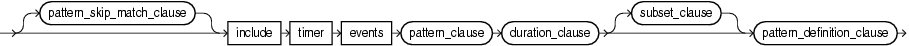## 15.10 SUBSET Clause

Using this clause, you can group together one or more correlation variables that are defined in the `DEFINE` clause. You can use this named subset in the `MEASURES` and `DEFINE` clauses just like any other correlation variable.

For example:

```SUBSET S1 = (Z,X)
```

The right-hand side of the subset (`(Z,X)`) is a comma-separated list of one or more correlation variables as defined in the `PATTERN` clause.

The left-hand side of the subset (`S1`) is the union of the correlation variables on the right-hand side.

You cannot include a subset variable in the right-hand side of a subset.

Figure 15-11 subset_clause::=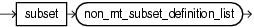Figure 15-12 non_mt_subset_definition_list::=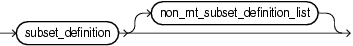Figure 15-13 subset_definition::=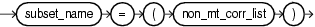Figure 15-14 subset_name::=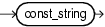Figure 15-15 non_mt_corr_list::=Consider the query `q55` inExample 15-1 and the data stream `S11` in Example 15-2. Stream `S11` has schema `(c1 integer, c2 integer)`. This example defines subsets `S1` through `S6`. This query outputs a match if the `c2` attribute values in the input stream form the shape of the English letter W. Now suppose we want to know the sum of the values of `c2` for those tuples which form the incrementing arms of this W shape. The correlation variable `X` represents tuples that are part of the first incrementing arm and `Z` represent the tuples that are part of the second incrementing arm. So we need some way to group the tuples that match both. Such a requirement can be captured by defining a `SUBSET` clause as the example shows.

Subset `S4` is defined as `(X,Z)`. It refers to the tuples in the input stream that match either `X` or `Z`. This subset is used in the `MEASURES` clause statement `sum(S4.c2) as sumIncrArm`. This computes the sum of the value of `c2` attribute in the tuples that match either `X` or `Z`. A reference to `S4.c2` in a `DEFINE` clause like `S4.c2 = 10` will refer to the value of `c2` in the latest among the last tuple that matched `X` and the last tuple that matched `Z`.

Subset `S6` is defined as `(Y)`. It refers to all the tuples that match correlation variable `Y`.

The query returns the stream.

Example 15-1 SUBSET Clause Query

```<query id="q55"><![CDATA[
select
T.firstW,
T.lastZ,
T.sumDecrArm,
T.sumIncrArm,
T.overallAvg
from
S11
MATCH_RECOGNIZE (
MEASURES
S2.c1 as firstW,
last(S1.c1) as lastZ,
sum(S3.c2) as sumDecrArm,
sum(S4.c2) as sumIncrArm,
avg(S5.c2) as overallAvg
PATTERN(A W+ X+ Y+ Z+)
SUBSET S1 = (Z) S2 = (A) S3 = (A,W,Y) S4 = (X,Z) S5 = (A,W,X,Y,Z) S6 = (Y)
DEFINE
W as W.c2 < prev(W.c2),
X as X.c2 > prev(X.c2),
Y as S6.c2 < prev(Y.c2),
Z as Z.c2 > prev(Z.c2)
) as T
]]></query>
```

Example 15-2 SUBSET Clause Example

```Timestamp   Tuple
1000        1,8
2000        2,8
3000        3,8
4000        4,6
5000        5,3
6000        6,7
7000        7,6
8000        8,2
9000        9,6
10000       10,2
11000       11,9
12000       12,9
13000       13,8
14000       14,5
15000       15,0
16000       16,9
17000       17,2
18000       18,0
19000       19,2
20000       20,3
21000       21,8
22000       22,5
23000       23,9
24000       24,9
25000       25,4
26000       26,7
27000       27,2
28000       28,8
29000       29,0
30000       30,4
31000       31,4
32000       32,7
33000       33,8
34000       34,6
35000       35,4
36000       36,5
37000       37,1
38000       38,7
39000       39,5
40000       40,8
41000       41,6
42000       42,6
43000       43,0
44000       44,6
45000       45,8
46000       46,4
47000       47,3
48000       48,8
49000       49,2
50000       50,5
51000       51,3
52000       52,3
53000       53,9
54000       54,8
55000       55,5
56000       56,5
57000       57,9
58000       58,7
59000       59,3
60000       60,3
```
```Timestamp   Tuple Kind  Tuple
9000:      +           3,9,25,13,5.428571
21000:      +           12,21,24,22,4.6
28000:      +           24,28,15,15,6.0
38000:      +           33,38,19,12,5.1666665
48000:      +           42,48,13,22,5.0
```

## 15.11 MATCH_RECOGNIZE Examples

The following examples illustrate basic `MATCH_RECOGNIZE` practices:

### 15.11.1 Pattern Detection

Consider the stock fluctuations that Figure 15-16 shows. This data can be represented as a stream of stock ticks (index number or time) and stock price. Figure 15-16 shows a common trading behavior known as a double bottom pattern between days 1 and 9 and between days 12 and 19. This pattern can be visualized as a W-shaped change in stock price: a fall (`X`), a rise (`Y`), a fall (`W`), and another rise (`Z`).

Figure 15-16 Pattern Detection: Double Bottom Stock Fluctuations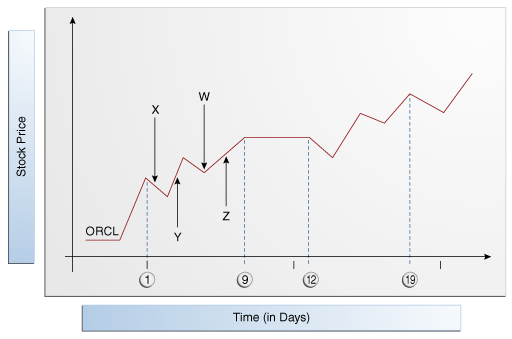Description of "Figure 15-16 Pattern Detection: Double Bottom Stock Fluctuations"

Example 15-3 shows a query `q` on stream `S2` of stock price events with schema `symbol`, `stockTick`, and `price`. This query detects double bottom patterns on the incoming stock trades using the `PATTERN` clause (`A W+ X+ Y+ Z+`). The correlation names in this clause are:

• `A`: corresponds to the start point of the double bottom pattern.

Because correlation name `A` is true for every input, it is not defined in the `DEFINE` clause. If you specify a correlation name that is not defined in the `DEFINE` clause, it is considered to be true for every input.

• `W`+: corresponds to the first decreasing arm of the double bottom pattern.

It is defined by `W.price < prev(W.price)`. This definition implies that the current price is less than the previous one.

• `X+`: corresponds to the first increasing arm of the double bottom pattern.

• `Y+`: corresponds to the second decreasing arm of the double bottom pattern.

• `Z+`: corresponds to the second increasing arm of the double bottom pattern.

Example 15-3 Pattern Detection

```<query id="q"><![CDATA[
SELECT
T.firstW,
T.lastZ
FROM
S2
MATCH_RECOGNIZE (
MEASURES
A.stockTick as firstW,
last(Z) as lastZ
PATTERN(A W+ X+ Y+ Z+)
DEFINE
W as W.price < prev(W.price),
X as X.price > prev(X.price),
Y as Y.price < prev(Y.price),
Z as Z.price > prev(Z.price)
) as T
WHERE
S2.symbol = "oracle"
]]></query>
```

### 15.11.2 Pattern Detection With PARTITION BY

Consider the stock fluctuations that Figure 15-17 shows. This data can be represented as a stream of stock ticks (index number or time) and stock price. In this case, the stream contains data for more than one stock ticker symbol. Figure 15-17 shows a common trading behavior known as a double bottom pattern between days 1 and 9 and between days 12 and 19 for stock BOFA. This pattern can be visualized as a W-shaped change in stock price: a fall (`X`), a rise (`Y`), a fall (`W`), and another rise (`Z`).

Figure 15-17 Pattern Detection With Partition By: Stock Fluctuations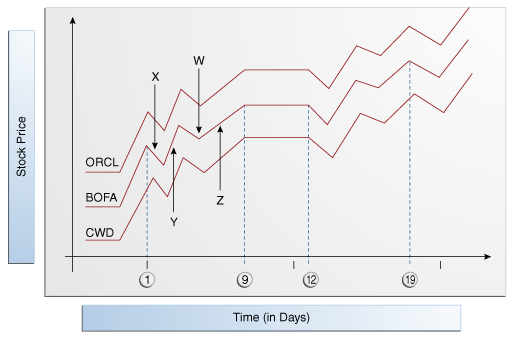Description of "Figure 15-17 Pattern Detection With Partition By: Stock Fluctuations"

Example 15-4 shows a query `q` on stream `S2` of stock price events with schema `symbol`, `stockTick`, and `price`. This query detects double bottom patterns on the incoming stock trades using the `PATTERN` clause (`A W+ X+ Y+ Z+`). The correlation names in this clause are:

• `A`: corresponds to the start point of the double bottom pattern.

• `W`+: corresponds to the first decreasing arm of the double bottom pattern as defined by `W.price < prev(W.price)`, which implies that the current price is less than the previous one.

• `X+`: corresponds to the first increasing arm of the double bottom pattern.

• `Y+`: corresponds to the second decreasing arm of the double bottom pattern.

• `Z+`: corresponds to the second increasing arm of the double bottom pattern.

The query partitions the input stream by stock ticker symbol using the `PARTITION BY` clause and applies this `PATTERN` clause to each logical stream.

Example 15-4 Pattern Detection With PARTITION BY

```<query id="q"><![CDATA[
SELECT
T.firstW,
T.lastZ
FROM
S2
MATCH_RECOGNIZE (
PARTITION BY
A.symbol
MEASURES
A.stockTick as firstW,
last(Z) as lastZ
PATTERN(A W+ X+ Y+ Z+)
DEFINE
W as W.price < prev(W.price),
X as X.price > prev(X.price),
Y as Y.price < prev(Y.price),
Z as Z.price > prev(Z.price)
) as T
]]></query>
```

### 15.11.3 Pattern Detection With Aggregates

Consider the query `q1` and the data stream `S`. Stream `S` has schema `(c1 integer)`. The query returns the stream.

```<query id="q1"><![CDATA[
SELECT
T.sumB
FROM
S
MATCH_RECOGNIZE (
MEASURES
sum(B.c1) as sumB
PATTERN(A B* C)
DEFINE
A as ((A.c1 < 50) AND (A.c1 > 35)),
B as B.c1 > avg(A.c1),
C as C.c1 > prev(C.c1)
) as T
]]></query>
```
```Timestamp   Tuple
1000       40
2000       52
3000       60
4000       58
5000       57
6000       56
7000       55
8000       59
9000       30
10000       40
11000       52
12000       60
13000       58
14000       57
15000       56
16000       55
17000       30
18000       10
19000       20
20000       30
21000       10
22000       25
23000       25
24000       25
25000       25
```
```Timestamp   Tuple
8000        338
12000       52
```

### 15.11.4 Pattern Detection With the WITHIN Clause

Consider the queries and the data stream `S`. Stream `S` has schema `(c1 integer, c2 integer)`. Table 15-3 compares the output of these queries.

```<query id="queryWithin"><![CDATA[
SELECT T.Ac2, T.Bc2, T.Cc2
FROM S
MATCH_RECOGNIZE(
MEASURES A.c2 as Ac2, B.c2 as Bc2, C.c2 as Cc2
PATTERN (A (B+ | C)) within 3000 milliseconds
DEFINE
A as A.c1=10 or A.c1=25,
B as B.c1=20 or B.c1=15 or B.c1=25,
C as C.c1=15
) as T
]]></query>
```
```<query id="queryWithinInclusive"><![CDATA[
SELECT T.Ac2, T.Bc2, T.Cc2
FROM S
MATCH_RECOGNIZE(
MEASURES A.c2 as Ac2, B.c2 as Bc2, C.c2 as Cc2
PATTERN (A (B+ | C)) within inclusive 3000 milliseconds
DEFINE
A as A.c1=10 or A.c1=25,
B as B.c1=20 or B.c1=15 or B.c1=25,
C as C.c1=15
) as T
]]></query>
```
```Timestamp   Tuple
1000       10,100
h 2000
3000       15,200
3000       20,300
4000       25,400
5000       20,500
6000       20,600
7000       35,700
8000       10,800
9000       15,900
h 11000
11000       20,1000
11000       50,1100
```

Table 15-3 WITHIN and WITHIN INCLUSIVE Query Output

Query queryWithin Query queryWithinInclusive
```Timestamp   Tuple Kind  Tuple
```
```3000:      +           100,300,
6000:      +           400,600,
9000:      +           800,900,
```
```Timestamp   Tuple Kind  Tuple
```
``` 4000:      +           100,400,
11000:      +           800,1000,
```

As Table 15-3 shows for the `queryWithin` query, the candidate match starts with the event at `TimeStamp=1000` and since the `WITHIN` clause duration is 3 seconds, the query will output the match only if it completes before the event at `TimeStamp=4000`. When the query receives the event at `TimeStampe=4000`, the longest match up to that point (since we are not using `ALL MATCHES`) is output. Note that although the event at `TimeStamp=4000` matches `B`, it is not included in the match. The next match starts with the event at `TimeStamp=4000` since that event also matches `A` and the previous match ends at `TimeStamp=3000`.

As Table 15-3 shows for the `queryWithinInclusive` query, the candidate match starts with the event at `TimeStamp=1000`. When the query receives the event at `TimeStamp=4000`, that event is included in the match because the query uses `WITHIN INCLUSIVE` and the event matches `B`. Note that although the event at `TimeStamp=5000` matches `B`, the pattern is not grown further since it exceeds the duration (3 seconds) measured from the start of the match (`TimeStamp=1000`). Since this match ends at `TimeStamp=4000` and we are not using `ALL MATCHES`, the next match does not start at `TimeStamp=4000`, even though it matches `A`.

### 15.11.5 Fixed Duration Non-Event Detection

Consider an object that moves among five different rooms. Each time it starts from room 1, it must reach room 5 within 5 minutes. Figure 15-18 shows the object's performance. This data can be represented as a stream of time and room number. Note that when the object started from room 1 at time 1, it reached room 5 at time 5, as expected. However, when the object started from room 1 at time 6, it failed to reach room 5 at time 11; it reached room 5 at time 12. When the object started from room 1 at time 15, it was in room 5 at time 20, as expected. However, when the object started from room 1 at time 23, it failed to reach room 5 at time 28; it reached room 5 at time 30. The successes at times 5 and 20 are considered events: the arrival of the object in room 5 at the appropriate time. The failures at time 11 and 28 are considered non-events: the expected arrival event did not occur. Using Oracle CQL, you can query for such non-events.

Figure 15-18 Fixed Duration Non-Event Detection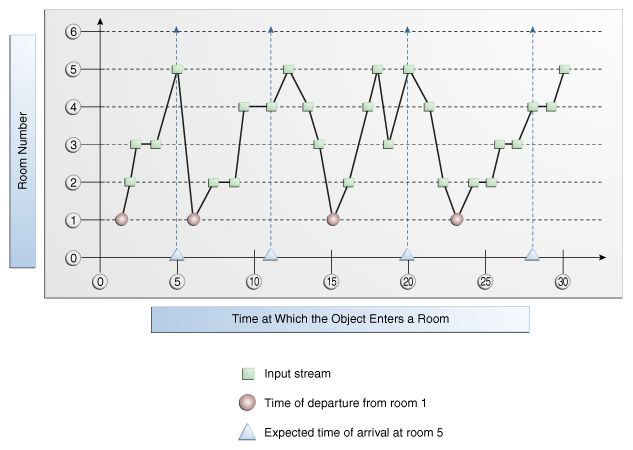Description of "Figure 15-18 Fixed Duration Non-Event Detection"

The following example shows query `q` on stream `S` (with schema `c1` integer representing room number) that detects these non-events. Each time the object fails to reach room 5 within 5 minutes of leaving room 1, the query returns the time of departure from room 1.

```<query id="q"><![CDATA[
select T.Atime FROM S
MATCH_RECOGNIZE(
MEASURES
A.ELEMENT_TIME as Atime
INCLUDE TIMER EVENTS
PATTERN (A B*)
DURATION 5 MINUTES
DEFINE
A as A.c1 = 1,
B as B.c1 != 5
) as T
]]></query>
```